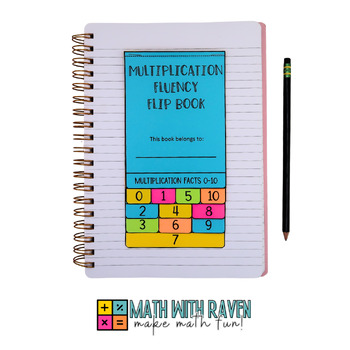# 3rd Grade Math Flip Book BundleSubject
Resource Type
Format
Zip (46 MB|5 FLIP BOOKS)
Standards
\$15.00
Bundle
List Price:
\$20.00
You Save:
\$5.00
\$15.00
Bundle
List Price:
\$20.00
You Save:
\$5.00

#### Also included in

1. This is not your average multiplication and division task card bundle. The resource also comes with and interactive notebook option with interactive notebook pockets and flip books for each volume. You can use the task cards in math centers, math rotations, small groups, for bell-ringers, etc... The
\$49.00
\$84.50
Save \$35.50

### Description

The bundle includes flip books that cover the strategies of multiplication, properties of multiplication, and the CUBES math strategy for word problems. Each flip book provides a reference for your math students that they can store in their interactive notebooks.

See the preview to view pictures of each flip book. See below for more information about each flip book.

Strategies of Multiplication Flip Book

In these flip books your students will learn the multiplication strategies to master multiplication fluency. Put them in interactive notebooks or just for reference. The flip books include strategies; arrays, grouping, repeated addition, number lines, and skip counting.

WHAT THEY COVER:

• Grouping
• Arrays
• Number Lines
• Skip Counting

**Please Notice: This resource has been updated and contains the NEW and OLD version of this flip book.

------------------------------------------------------------------------------------------------------------------------

Multiplication Flip Book Facts 0-10 & 0-12

This multiplication fact flip book introduces facts in the most simple way. Your third-grade math students will say the multiplication sentence and then write the multiplication sentence.

The way the facts are introduced in the flip book are the order in which they should be taught:

0, 1, 5, 10

2, 4, 8

3, 6, 9

7

➡Recently added by request version 0-12:

0, 1, 5, 10

2, 4, 8

3, 6, 9

7, 11, 12

------------------------------------------------------------------------------------------------------------------------

Properties of Multiplication Flip Book

These properties of multiplication flip books for third-grade math are great for reinforcing your students' knowledge on the properties of multiplication. The flip books include the associative property, distributive property, and commutative property of multiplication. The flip books align with CCSS 3.OA.B.5 for third-grade math.

Flip Book Includes:

• Associative Property description, definition, & example
• Commutative Property description, definition, & example
• Distributive Property description, definition, & example

Each property comes with a workable problem so your student can interact with the flip book.

Print on colored paper to make your flip books REALLY pop.

------------------------------------------------------------------------------------------------------------------------

CUBES Math Strategy Flip Book

Help your students with those tough math word problems with this engaging CUBES math strategy flip book. The flip book provides your students with tips to break down intimidating word problems and find success.

These flip books fit perfectly in interactive math notebooks!

------------------------------------------------------------------------------------------------------------------------

Division Flip Book Facts 1-10

This division fact flip book introduces facts in the most simple way. Your third-grade math students will say the division sentence and then write the division sentence.

The way the facts are introduced in the flip book is the order in which I prefer to teach them:

1, 5, 10

2, 4, 8

3, 6, 9

7

★★★★★★★★★★★★★★★★★★★★★★★★★★★★★★★★★★★★★★★★★★★★★★★★★★★★

LET'S STAY CONNECTED!

Total Pages
5 FLIP BOOKS
N/A
Teaching Duration
N/A
Report this Resource to TpT
Reported resources will be reviewed by our team. Report this resource to let us know if this resource violates TpT’s content guidelines.

### Standards

to see state-specific standards (only available in the US).
Solve multistep word problems posed with whole numbers and having whole-number answers using the four operations, including problems in which remainders must be interpreted. Represent these problems using equations with a letter standing for the unknown quantity. Assess the reasonableness of answers using mental computation and estimation strategies including rounding.
Multiply or divide to solve word problems involving multiplicative comparison, e.g., by using drawings and equations with a symbol for the unknown number to represent the problem, distinguishing multiplicative comparison from additive comparison.
Solve two-step word problems using the four operations. Represent these problems using equations with a letter standing for the unknown quantity. Assess the reasonableness of answers using mental computation and estimation strategies including rounding.
Fluently multiply and divide within 100, using strategies such as the relationship between multiplication and division (e.g., knowing that 8 × 5 = 40, one knows 40 ÷ 5 = 8) or properties of operations. By the end of Grade 3, know from memory all products of two one-digit numbers.
Apply properties of operations as strategies to multiply and divide. Examples: If 6 × 4 = 24 is known, then 4 × 6 = 24 is also known. (Commutative property of multiplication.) 3 × 5 × 2 can be found by 3 × 5 = 15, then 15 × 2 = 30, or by 5 × 2 = 10, then 3 × 10 = 30. (Associative property of multiplication.) Knowing that 8 × 5 = 40 and 8 × 2 = 16, one can find 8 × 7 as 8 × (5 + 2) = (8 × 5) + (8 × 2) = 40 + 16 = 56. (Distributive property.)